# Summation Notation and Mathematical Series

An error occurred trying to load this video.

Try refreshing the page, or contact customer support.

Coming up next: How to Calculate an Arithmetic Series

### You're on a roll. Keep up the good work!

Replay
Your next lesson will play in 10 seconds
• 0:06 Defining a Series
• 0:49 Using Sigma
• 3:04 Building a Formula
• 5:25 Lesson Summary
Save Save

Want to watch this again later?

Timeline
Autoplay
Autoplay
Speed Speed

#### Recommended Lessons and Courses for You

Lesson Transcript
Instructor
Jeff Calareso

Jeff teaches high school English, math and other subjects. He has a master's degree in writing and literature.

Expert Contributor
Kathryn Boddie

Kathryn earned her Ph.D. in Mathematics from UW-Milwaukee in 2019. She has over 10 years of teaching experience at high school and university level.

The capital Greek letter sigma might be most recognizable as a common symbol in a fraternity or sorority, but it's used for some pretty cool math tricks too. In this lesson, learn how to use sigma for things like determining how many seats are in Michigan Stadium.

## Understanding a Number Series

So now that we know what a sequence is, we can actually start putting them to use. The most basic thing we can do with a sequence is to take all its entries, and add them up. When you do this, you turn something like 5, 6, 7, 8, which would be a sequence, into 5 + 6 + 7 + 8, which is now a series.

So instead of simply being able to say things like, 'The 34th row in Michigan Stadium has 167 seats,' we can instead say things like, 'There are 3,434 seats in the first 34 rows of one section of Michigan Stadium.'

## Series Notation Using Sigma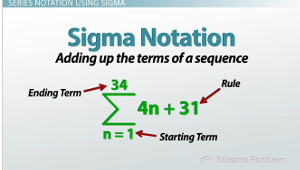I got that number simply by doing 35, because there were 35 seats in the first row, plus 39, because there are 4 more in each row, plus 43, plus 47, all the way out to plus 163, plus 167, because that's how many seats there would be in the 34th row. If you add all those numbers up, you get 3,434.

The thing is, I didn't sit down with my calculator and put in 35 + 39 + 43 all the way to 167, because that would've taken me a long time. Surprise, surprise, there's a shortcut. Now, we're going to learn a shortcut for how to actually add those numbers up in a later lesson. In this lesson, we're simply going to learn the way of writing that series out without having to use this '…' (35 + 39 + 43 + … + 167).

This is where the Greek letter sigma comes in. Anytime you see this letter in math, it's implying that we'll be taking a series, which means that we're just adding up the terms of a sequence.

What goes underneath the letter will tell you where we start the sequence. So, it tells you the starting term, n = something. The number on the top is where we end the series. The only thing missing is the rule, and that goes directly to the right of the sigma. What we have after we fill all those things in is either called sigma notation or summation notation.

Therefore, if I was going to express this series that tells us how many seats are in the first 34 rows of Michigan Stadium, I would draw my Greek letter sigma in. I would put n = 1 underneath is, because we're starting with the first row. I would put a 34 on top of it, because we're adding through row 34. And, I would put 4n + 31 next to the sigma. That's my rule because it goes up by 4 seats each time we add a row and if there was a 0 row, it would have 31 seats.

## Building a Series Formula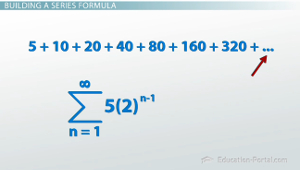So, let's quickly look at another random example. Let's say that we're asked to express the series 5 + 10 + 20 +40 + 80 + 160 + 320 + … using summation notation.

To unlock this lesson you must be a Study.com Member.

## Extra Practice with Summation Notation

In the following examples, students will evaluate the sums of finite series written in summation notation, as well as translate series into summation notation. These skills help emphasize the difference between a sequence and a series and develop pattern recognition.

## Practice Problems

1) Evaluate the sum: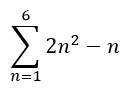2) Evaluate the sum: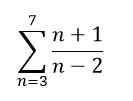3) Write the following series in summation notation: 40 + 20 + 10 + 5 + ...

4) Write the following series in summation notation: -1 + 4 - 9 + 16 - 25 + 36 - ....

## Solutions

1) To evaluate the sum, begin by substituting n = 1 into the rule, then add the result from substituting n = 2 into the rule, and so on, ending with substituting n = 6 into the rule.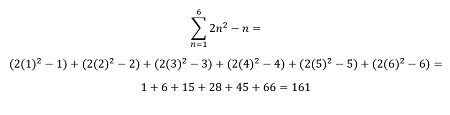2) To evaluate the sum, begin by substituting n = 3 into the rule, then add the result from substituting n = 4 into the rule, and so on, ending with substituting n = 7 into the rule.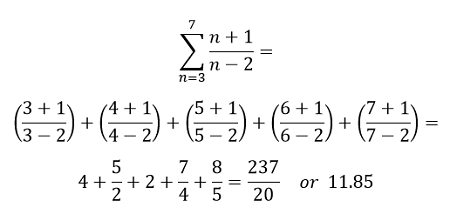3) This is a geometric series in which each term is multiplied by 1/2 to find the next term. Using the general form for a geometric series which starts at 40 and has r = 1/2, we have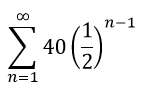4) This is not a geometric series, but we can still find a pattern. The terms in the series are alternating between positive and negative. This behavior can be represented using powers of -1. The terms which are alternating are also perfect squares, since 1 = 1^2, 4 = 2^2, 9 = 3^2, and so on. So, inside our summation notation, we need powers of -1, and we need n^2. Putting it together, we have: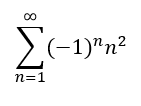### Register to view this lesson

Are you a student or a teacher?

#### See for yourself why 30 million people use Study.com

##### Become a Study.com member and start learning now.
Back
What teachers are saying about Study.com

### Earning College Credit

Did you know… We have over 200 college courses that prepare you to earn credit by exam that is accepted by over 1,500 colleges and universities. You can test out of the first two years of college and save thousands off your degree. Anyone can earn credit-by-exam regardless of age or education level.